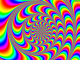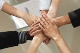# GridDateTimeColumn DataFormatString not working from codebehind

GridTop achievementsRank 1
Daniele asked on 22 Jul 2014, 11:05 AM
Hi all,
I need to add a GridDateTimeColumn to show and allow the user change a time, so I need to display data in format HH:ss .
I am trying to do this using DataFormatString property but it doesn't work: I always see the standard format yyyy/MM/dd HH:mm:ss .

Here is my code:

GridDateTimeColumn col = new GridDateTimeColumn();
col.PickerType = GridDateTimeColumnPickerType.TimePicker;
col.DataField = "FIELD";
col.DataType = typeof(DateTime);
col.DataFormatString = "{0:HH mm}";

Can anyone help me?

## 3 Answers, 1 is accepted

0Princy
Top achievementsRank 1
answered on 22 Jul 2014, 12:22 PM
Hi Daniele,

Your code looks fine, it worked fine at my end. Please try the sample code snippet and check if it helps.

C#:
`RadGrid rgrdSample;`
`protected` `void` `Page_Init(``object` `source, System.EventArgs e)`
`{`
`    ``rgrdSample = ``new` `RadGrid();`
`    ``rgrdSample.ID = ``"rgrdSample"``;`
`    ``rgrdSample.MasterTableView.DataKeyNames = ``new` `string``[] { ``"OrderID"` `};   `
`    ``rgrdSample.AllowPaging = ``true``;`
`    ``rgrdSample.AutoGenerateColumns = ``false``;`
`    ``rgrdSample.AllowSorting = ``true``;   `
`    ``rgrdSample.NeedDataSource += ``new` `GridNeedDataSourceEventHandler(RadGrid1_NeedDataSource);`
`    ``rgrdSample.PreRender += ``new` `EventHandler(rgrdSample_PreRender);`

`    ``GridBoundColumn boundColumn;`
`    ``boundColumn = ``new` `GridBoundColumn();`
`    ``boundColumn.DataField = ``"OrderID"``;`
`    ``boundColumn.HeaderText = ``"OrderID"``;  `
`    ``rgrdSample.MasterTableView.Columns.Add(boundColumn);`

`    ``boundColumn = ``new` `GridBoundColumn();`
`    ``boundColumn.DataField = ``"ShipCountry"``;`
`    ``boundColumn.HeaderText = ``"ShipCountry"``;`
`    ``boundColumn.SortExpression = ``"ShipCountry"``;`
`    ``rgrdSample.MasterTableView.Columns.Add(boundColumn);`

`    ``GridDateTimeColumn datecolumn = ``new` `GridDateTimeColumn();`
`    ``datecolumn.PickerType = GridDateTimeColumnPickerType.TimePicker;`
`    ``datecolumn.DataField = ``"OrderDate"``;`
`    ``datecolumn.HeaderText = ``"OrderDate"``;`
`    ``datecolumn.DataType = Type.GetType(``"System.DateTime"``);`
`    ``datecolumn.DataFormatString = ``"{0:HH:mm}"``;`
`    ``datecolumn.UniqueName = ``"OrderDate"``;`
`    ``rgrdSample.MasterTableView.Columns.Add(datecolumn);  `

`    ``this``.PlaceHolder1.Controls.Add(rgrdSample);    `
`}`
`    `
`//Another way is you can try to set in PreRender event if the above doesn't work`
`void` `rgrdSample_PreRender(``object` `sender, EventArgs e)`
`{`
`    ``GridBoundColumn col;`
`    ``col = (GridBoundColumn)rgrdSample.MasterTableView.GetColumn(``"OrderDate"``);`
`    ``col.DataType = Type.GetType(``"System.DateTime"``);`
`    ``col.DataFormatString = ``"{0:HH:mm}"``;`
`    ``rgrdSample.Rebind();`
`}`

`void` `RadGrid1_NeedDataSource(``object` `sender, GridNeedDataSourceEventArgs e)`
`{`
`    ``rgrdSample.DataSource = GetDataTable(``"SELECT  *  FROM Orders"``);`
`}`

`public` `DataTable GetDataTable(``string` `query)`
`{`
`    ``String ConnString = ConfigurationManager.ConnectionStrings[``"ConnectionString"``].ConnectionString;`
`    ``SqlConnection conn = ``new` `SqlConnection(ConnString);`
`    ``SqlDataAdapter adapter = ``new` `SqlDataAdapter();`
`    ``adapter.SelectCommand = ``new` `SqlCommand(query, conn);`
`    ``DataTable myDataTable = ``new` `DataTable();`
`    ``conn.Open();`
`    ``try`
`    ``{`
`        ``adapter.Fill(myDataTable);`
`    ``}`
`    ``finally`
`    ``{`
`        ``conn.Close();`
`    ``}`
`    ``return` `myDataTable;`
`}`

Thanks,
Princy
0Daniele
Top achievementsRank 1
answered on 22 Jul 2014, 12:50 PM
I tried to add formatting in the PreRender event, but always the same.

To be sure that what I am doing takes effect, I tried the following in my code:
col.DataFormatString = "xx{0:HH mm}";
In this way I can see the following (for example):
xx2014/01/01 07:30:00
That means that the formatting that I am setting takes somehow effect on rendering.

But I cannot understand why the part in brackets takes no effect.
0Daniele
Top achievementsRank 1
answered on 22 Jul 2014, 01:54 PM
I solved it!!
The datasource of my RadGrid is a DataTable which is created dinamically, too.
Even if in my column I was adding DateTime values, I had not specified the type of the column:
[...]
[...]
row["date"] = DateTime.Now;
[...]

Specifyng tha type of data in this datasource solved the problem:
[...]
[...]
row["date"] = DateTime.Now;
[...]

Thank you all!!!
Tags
GridDaniele
Top achievementsRank 1Rank 1Rank 1